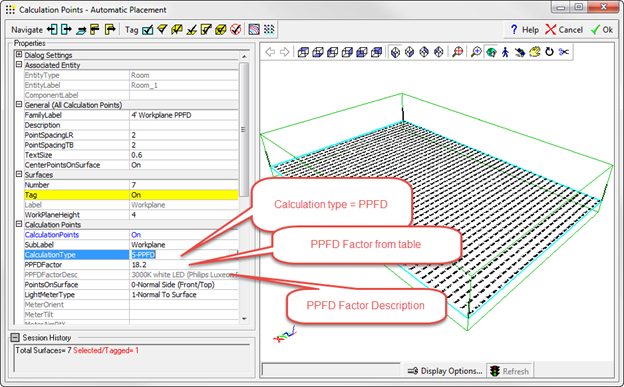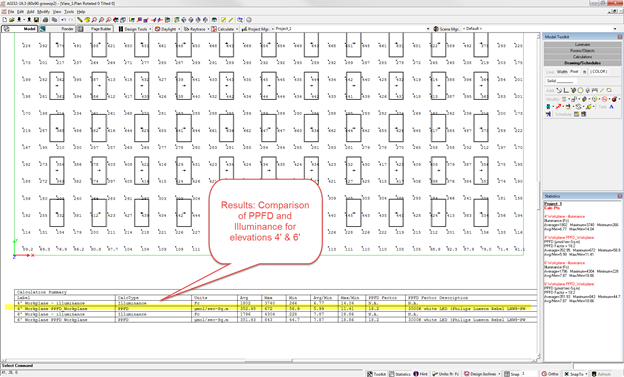The equivalent to illuminance (fc or lux) for plants is Photosynthetic Photon Flux Density. Specifying PPFD as a calculation metric in AGi32 is performed by selecting a specific calculation type (like illuminance or luminance) for the calculation point grid rather than as a light source characteristic. This assumes that all sources contributing to the calculation grid have the same PPFD Factor.

Calculation points must be placed on a surface or on an imaginary work plane. PPFD is then selected as the calculation type and the appropriate PPFD Factor either selected from the table, or, if known, entered in the field provided. AGi32 will use the PPFD Factor to convert illuminance to PPFD in micromoles per second per square meter (μmol/s-m2).

To specify PPFD calculations in AGi32:

1. Begin with a Room or Object that will have the calculation points in or on it. Initiate the Automatic Placement command.
1. Navigate to the Workplane or Tag the surface(s) where you wish to place the points.
1. Set the Point Spacing.
1. For the Calculation Type, select 5- PPFD (Figure 1).
1. For the PPFD Factor, click in the field to see the ellipsis (...) button, and then click on that button to open the PPFD Factors dialog.
1. Select one of the listed light sources, or add your own to the table by entering a new source name in the blank field at the bottom (marked with an asterisk) as well as a PPFD Factor (the conversion factor) in the second column (Figure 1).Figure 1

1. Click OK to return to the Automatic Placement dialog. The PPFD Factor and the source description will appear below the Calculation Type (5- PPFD).
1. Click OK to complete the command. The points will be placed as specified.
2. Calculate the results (Figure 2).Figure 2